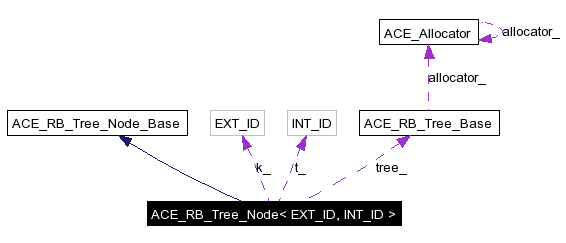# ACE_RB_Tree_Node< EXT_ID, INT_ID > Class Template Reference

Implements a node in a Red-Black Tree ADT. More...

`#include <RB_Tree.h>`

Inheritance diagram for ACE_RB_Tree_Node< EXT_ID, INT_ID >:[legend]
Collaboration diagram for ACE_RB_Tree_Node< EXT_ID, INT_ID >:[legend]
List of all members.

## Public Member Functions

ACE_RB_Tree_Node (const EXT_ID &k, const INT_ID &t, const ACE_RB_Tree_Base &tree)
Constructor.
~ACE_RB_Tree_Node (void)
Destructor.
EXT_ID & key (void)
Key accessor.
INT_ID & item (void)
Item accessor.
void color (RB_Tree_Node_Color c)
Set color of the node.
RB_Tree_Node_Color color (void)
Get color of the node.
ACE_RB_Tree_Node< EXT_ID,
INT_ID > *
parent (void)
Accessor for node's parent pointer.
void parent (ACE_RB_Tree_Node< EXT_ID, INT_ID > *p)
Mutator for node's parent pointer.
ACE_RB_Tree_Node< EXT_ID,
INT_ID > *
left (void)
Accessor for node's left child pointer.
void left (ACE_RB_Tree_Node< EXT_ID, INT_ID > *l)
Mutator for node's left child pointer.
ACE_RB_Tree_Node< EXT_ID,
INT_ID > *
right (void)
Accessor for node's right child pointer.
void right (ACE_RB_Tree_Node< EXT_ID, INT_ID > *r)
Mutator for node's right child pointer.

## Private Attributes

EXT_ID k_
The key.
INT_ID t_
The item.
RB_Tree_Node_Color color_
Color of the node.
ACE_RB_Tree_Node< EXT_ID,
INT_ID > *
parent_
Pointer to node's parent.
ACE_RB_Tree_Node< EXT_ID,
INT_ID > *
left_
Pointer to node's left child.
ACE_RB_Tree_Node< EXT_ID,
INT_ID > *
right_
Pointer to node's right child.
const ACE_RB_Tree_Basetree_
Pointer to tree base (to get the allocator).

## Detailed Description

### template<class EXT_ID, class INT_ID> class ACE_RB_Tree_Node< EXT_ID, INT_ID >

Implements a node in a Red-Black Tree ADT.

## Constructor & Destructor Documentation

 template ACE_RB_Tree_Node< EXT_ID, INT_ID >::ACE_RB_Tree_Node ( const EXT_ID & k, const INT_ID & t, const ACE_RB_Tree_Base & tree )
 Constructor.

 template ACE_RB_Tree_Node< EXT_ID, INT_ID >::~ACE_RB_Tree_Node ( void )
 Destructor.

## Member Function Documentation

 template ACE_INLINE ACE_RB_Tree_Node_Base::RB_Tree_Node_Color ACE_RB_Tree_Node< EXT_ID, INT_ID >::color ( void )
 Get color of the node.

 template ACE_INLINE void ACE_RB_Tree_Node< EXT_ID, INT_ID >::color ( RB_Tree_Node_Color c )
 Set color of the node.

 template ACE_INLINE INT_ID & ACE_RB_Tree_Node< EXT_ID, INT_ID >::item ( void )
 Item accessor.

 template ACE_INLINE EXT_ID & ACE_RB_Tree_Node< EXT_ID, INT_ID >::key ( void )
 Key accessor.

 template ACE_INLINE void ACE_RB_Tree_Node< EXT_ID, INT_ID >::left ( ACE_RB_Tree_Node< EXT_ID, INT_ID > * l )
 Mutator for node's left child pointer.

 template ACE_INLINE ACE_RB_Tree_Node< EXT_ID, INT_ID > * ACE_RB_Tree_Node< EXT_ID, INT_ID >::left ( void )
 Accessor for node's left child pointer.

 template ACE_INLINE void ACE_RB_Tree_Node< EXT_ID, INT_ID >::parent ( ACE_RB_Tree_Node< EXT_ID, INT_ID > * p )
 Mutator for node's parent pointer.

 template ACE_INLINE ACE_RB_Tree_Node< EXT_ID, INT_ID > * ACE_RB_Tree_Node< EXT_ID, INT_ID >::parent ( void )
 Accessor for node's parent pointer.

 template ACE_INLINE void ACE_RB_Tree_Node< EXT_ID, INT_ID >::right ( ACE_RB_Tree_Node< EXT_ID, INT_ID > * r )
 Mutator for node's right child pointer.

 template ACE_INLINE ACE_RB_Tree_Node< EXT_ID, INT_ID > * ACE_RB_Tree_Node< EXT_ID, INT_ID >::right ( void )
 Accessor for node's right child pointer.

## Member Data Documentation

 template RB_Tree_Node_Color ACE_RB_Tree_Node< EXT_ID, INT_ID >::color_` [private]`
 Color of the node.

 template EXT_ID ACE_RB_Tree_Node< EXT_ID, INT_ID >::k_` [private]`
 The key.

 template ACE_RB_Tree_Node* ACE_RB_Tree_Node< EXT_ID, INT_ID >::left_` [private]`
 Pointer to node's left child.

 template ACE_RB_Tree_Node* ACE_RB_Tree_Node< EXT_ID, INT_ID >::parent_` [private]`
 Pointer to node's parent.

 template ACE_RB_Tree_Node* ACE_RB_Tree_Node< EXT_ID, INT_ID >::right_` [private]`
 Pointer to node's right child.

 template INT_ID ACE_RB_Tree_Node< EXT_ID, INT_ID >::t_` [private]`
 The item.

 template const ACE_RB_Tree_Base* ACE_RB_Tree_Node< EXT_ID, INT_ID >::tree_` [private]`
 Pointer to tree base (to get the allocator).

The documentation for this class was generated from the following files:
Generated on Sat Aug 6 03:03:44 2005 for ACE by1.3.9.1